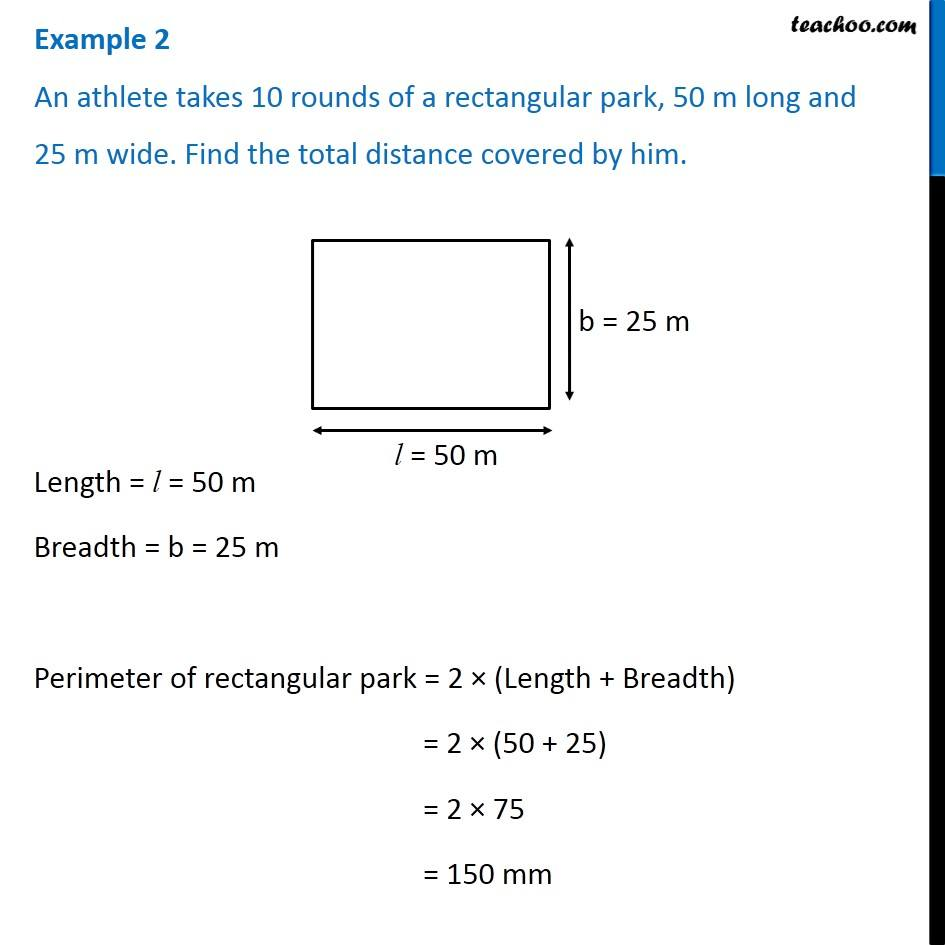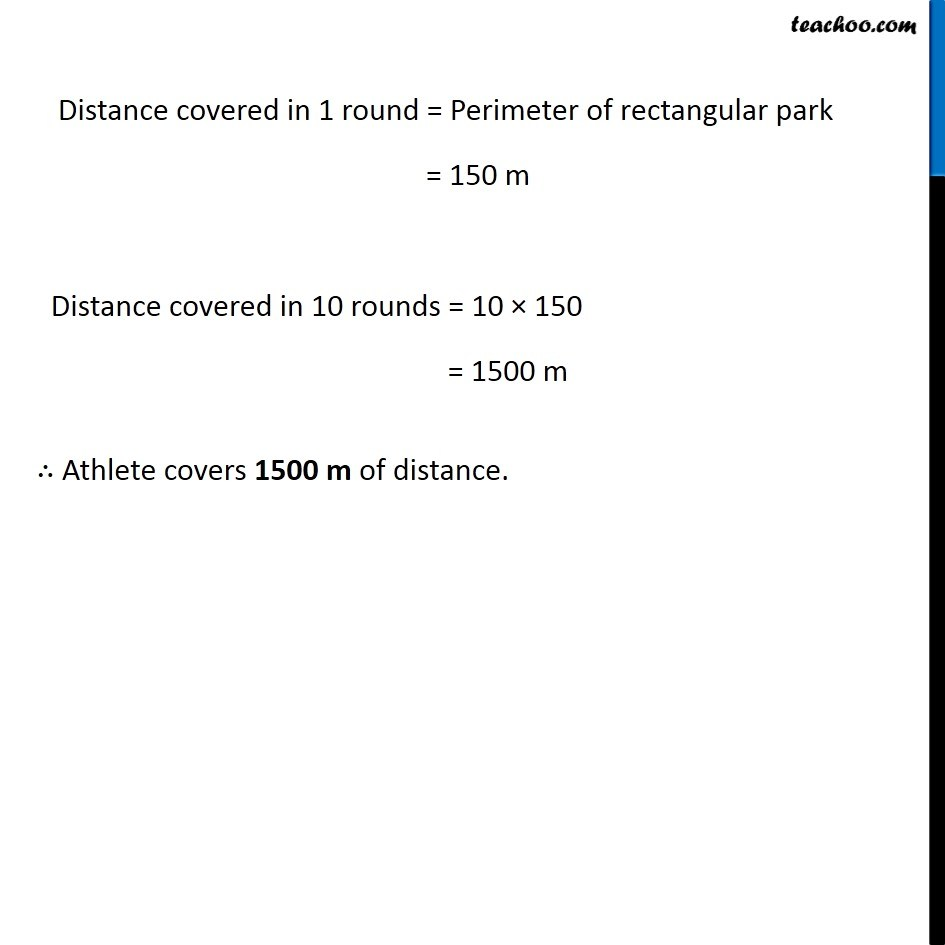Examples

Chapter 10 Class 6 Mensuration
Serial order wise### Transcript

Example 2 An athlete takes 10 rounds of a rectangular park, 50 m long and 25 m wide. Find the total distance covered by him. Length = l = 50 m Breadth = b = 25 m Perimeter of rectangular park = 2 × (Length + Breadth) = 2 × (50 + 25) = 2 × 75 = 150 mm Distance covered in 1 round = Perimeter of rectangular park = 150 m Distance covered in 10 rounds = 10 × 150 = 1500 m ∴ Athlete covers 1500 m of distance.